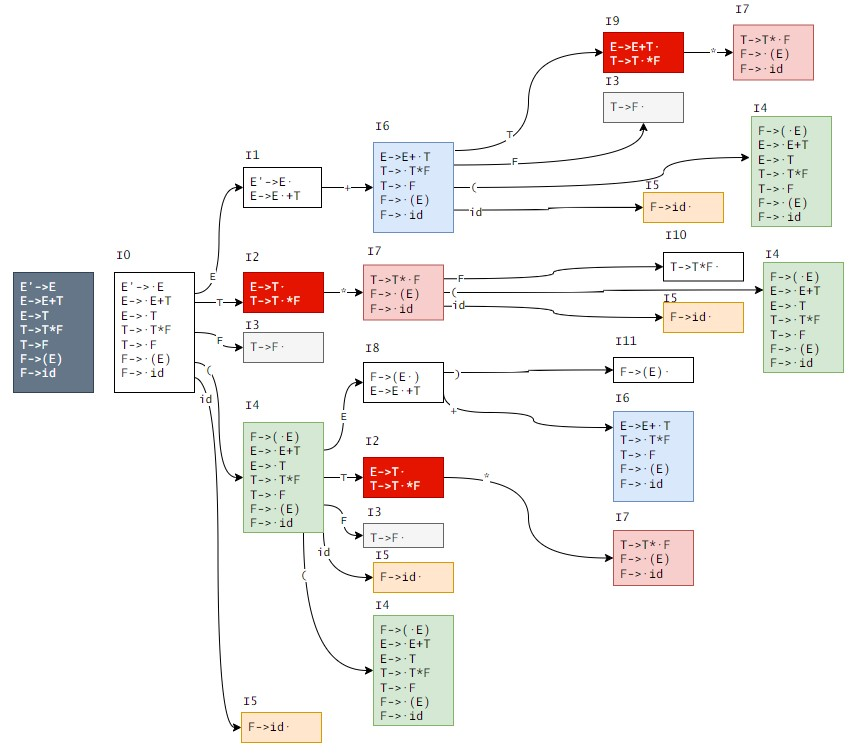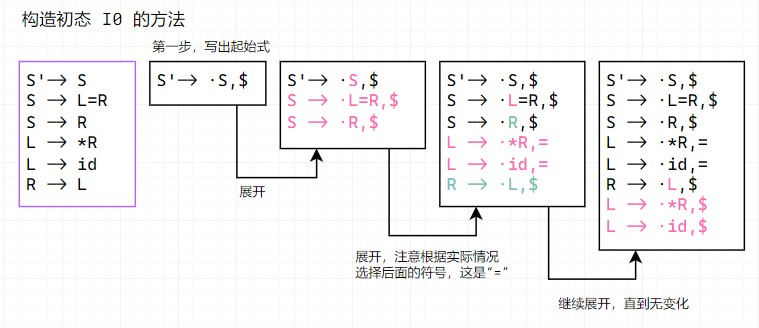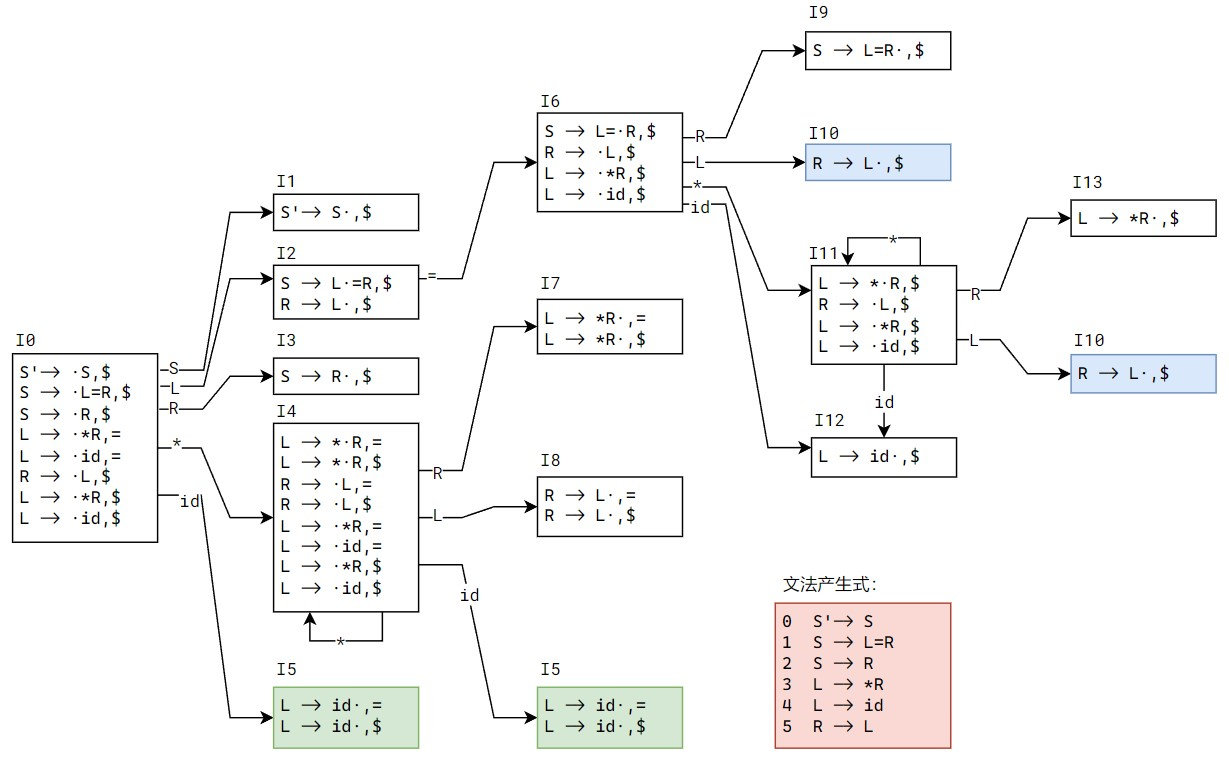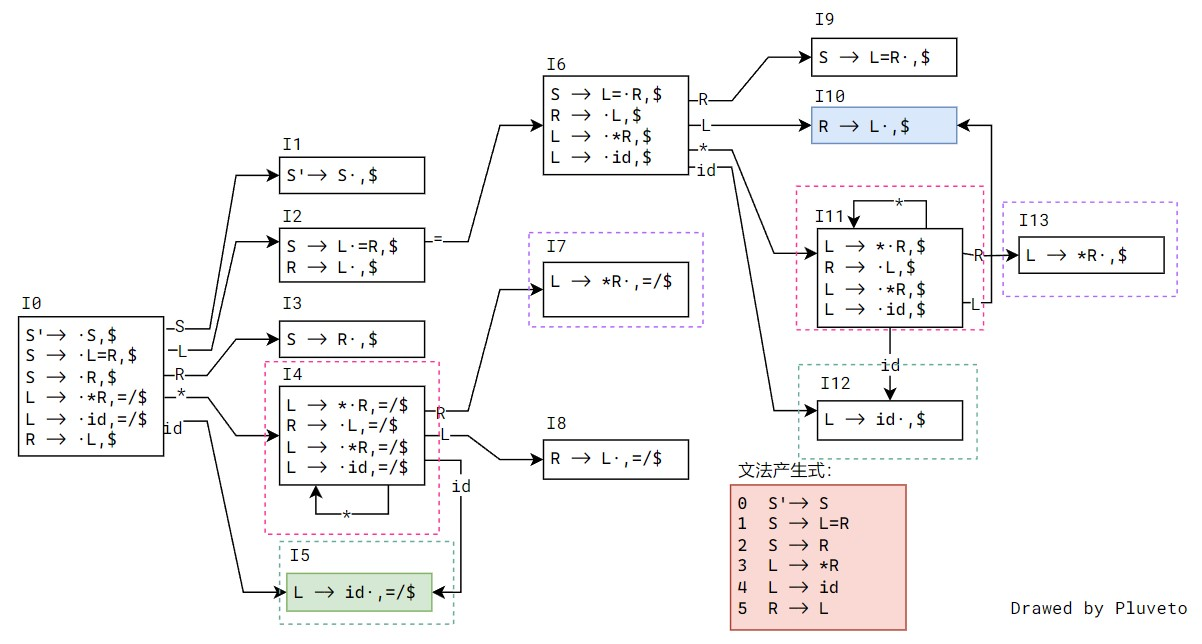## LL(1)

• First 可以有 $\varepsilon$ ，Follow 不能有。

（1）消除左递归得到：$L' \to EL' \mid \varepsilon , L = EL'$

$E \rightarrow A \mid B$ $A \rightarrow$ num $\mid$ id $B \rightarrow(L)$ $L' \to EL' \mid \varepsilon$ $L = EL'$

（2）

First
Follow

Symbol Nullable First Set Follow Set
$E \rightarrow A \mid B$ num id ( $ num id ($A \rightarrow$num$\mid$id num id $ num id (
$B \rightarrow(L)$ ( $ num id ($L' \to EL' \mid \varepsilon $T num id ( ε )$L \to EL'$num id ( ) （3）LL (1) 文法的判断 1. 根据产生式判断。对任意产生式$A \to a \mid b$，服从于： •$\operatorname{First} ( a ) \cap \operatorname{First} ( b ) = \emptyset $• 若$b$可致空，则$\operatorname {First}(a) \cap \operatorname {Follow}(A) = \emptyset $2. 根据分析表判断。如果 LL (1) 分析表无二义性，则文法是 LL (1) 文法。 方法 1 实际上是说： • 对于产生式的各个分支，一定能通过第一个词符判断选择哪个分支。 • 对于含有自指的分支，一定能通过第一个词符判断是否继续自指。 对于本题， 1. 就$E \rightarrow A \mid B$而言，$A,B$First Set 交集为空。 2. 就$L' \to EL' \mid \varepsilon $而言，First (EL') 与 Follow (L') 交集为空。 所以是 LL (1) 文法。 分析表的构造 Symbol num id ( ) $
$E$ $E \to A$ $E \to A$ $E \to B$
$A$ $A \to \text{num}$ $A\to \text{id}$
$B$ $B \to (L)$
$L$ $L \to EL'$ $L \to EL'$ $L \to EL'$
$L'$ $L' \to EL'$ $L' \to EL'$ $L' \to EL'$ $L'\to \varepsilon$

（4）(a (b (2))(c)) 的预测分析过程

Step Stack Input Output Annotation
1 $E (a (b (2))(c))$ $E \to B$ 栈顶是 (，查表，采用 $E \to B$ 规约
2 $B (a(b(2))(c))$ $B \to (L)$
3 $) L ( (a (b (2))(c))$ (L) 从右到左压栈
4 $)L a(b(2))(c))$
5 $)L a(b(2))(c))$ $L \to EL'$
6 $)L&#x27;E a(b(2))(c))$ $E \to A$
7 $)L&#x27;A a(b(2))(c))$ $A\to \text{id}$
8 $)L&#x27;id a(b(2))(c))$
9 $)L&#x27; (b(2))(c))$ $L' \to EL'$
10 $)L&#x27;E (b(2))(c))$ $E \to B$
11 $)L&#x27;B (b(2))(c))$ $B \to (L)$
12 $)L&#x27;)L( (b(2))(c))$
13 $)L&#x27;)L b(2))(c))$ $L \to EL'$
14 $)L&#x27;)L&#x27;E b(2))(c))$ $E \to A$
15 $)L&#x27;)L&#x27;A b(2))(c))$ $A\to \text{id}$
16 $)L&#x27;)L&#x27;id b(2))(c))$
17 $)L&#x27;)L&#x27; (2))(c))$
18 $)L&#x27;)L&#x27; (2))(c))$ $L' \to EL'$
19 $)L&#x27;)L&#x27;E (2))(c))$ $E \to B$
20 $)L&#x27;)L&#x27;B (2))(c))$ $B \to (L)$
21 $)L&#x27;)L&#x27;)L( (2))(c))$
22 $)L&#x27;)L&#x27;)L 2))(c))$
23 $)L&#x27;)L&#x27;)L 2))(c))$ $L \to EL'$
24 $)L&#x27;)L&#x27;)L&#x27;E 2))(c))$ $E \to A$
25 $)L&#x27;)L&#x27;)L&#x27;A 2))(c))$ $A \to \text{num}$
26 $)L&#x27;)L&#x27;)L&#x27;num 2))(c))$
27 $)L&#x27;)L&#x27;)L&#x27; ))(c))$
28 $)L&#x27;)L&#x27;)L&#x27; ))(c))$ $L' \to \varepsilon$
29 $)L&#x27;)L&#x27;) ))(c))$
30 $)L&#x27;)L&#x27; )(c))$ $L' \to \varepsilon$
31 $)L&#x27;) )(c))$
32 $)L&#x27; (c))$ $L' \to EL'$
33 $)L&#x27;E (c))$ $E \to B$
34 $)L&#x27;B (c))$ $B \to (L)$
35 $)L&#x27;)L( (c))$
36 $)L&#x27;)L c))$ $L \to EL'$
37 $)L&#x27;)L&#x27;E c))$ $E \to A$
38 $)L&#x27;)L&#x27;A c))$ $A\to \text{id}$
39 $)L&#x27;)L&#x27;id c))$
40 $)L&#x27;)L&#x27; ))$ $L' \to \varepsilon$
41 $)L&#x27;) ))$
42 $)L&#x27; )$ $L' \to \varepsilon$
43 $) )$
44 $ $

## LR(0)

E'->E
E->E+T
E->T
T->T*F
T->F
F->(E)
F->id## LR(1)

$S \rightarrow L=R$ $S \rightarrow R$ $L \rightarrow * R$ $L \rightarrow \mathbf{i d}$ $R \rightarrow L$

$S' \to S$ $S \rightarrow L=R$ $S \rightarrow R$ $L \rightarrow * R$ $L \rightarrow \mathbf{i d}$ $R \rightarrow L$

$S' \to S$ $S$ * id $S$ =  $$S \to L=R \mid RL,R$* id$R$=  $
$L\to\star R\mid\text{id}$ * id $R$ =  $$R \to LL$* id$L$=  $Ii                  Ij
+-------------+     +-------------+
| A -> c ·, d |     | A -> c ·, e |
| B -> c ·, e |     | B -> c ·, d |
+-------------+     +-------------+